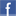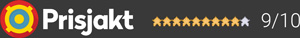#### Inga produkter tillagda!

Lägg en produkt i varukorgen så ser det lite roligare ut här ;)

#### Varukorg

0 kr12 990 kr/stLeverans 2-5 dagar ()
Fri frakt!

Läckert stereo-slutsteg från Advance Paris (Acoustic) på 2x160W

New schematics, same power transistors as MAA1000, new power supply, more power, more music, more modern cosmetics.

- X-A160EVO amplifier consists of a partioned ultra rigid chassis, providing effective shielding, which eliminates any risk of interference betwwen the different circuits of the device.

- The use of "Audiophile" components is the warranty of a detailed and subtlety reproduction of your favourite music.

- The high power of the power supply, combined with the « high current »power transistors (NJW0281G - NJW0302G) provides a powerful dynamic.

- The X-A160EVO provides a power of 160 W per channel in class AB, a switch on the rear panel allows to deliver the first watts in Class A. Beyond this limit, the amplifier commutes automatically in Class AB.

Specifications:

Power output 8 Ω : 2 X 160 W RMS
Power output 4 Ω : 2 X 240 W RMS
Frequency response : 10 Hz - 80 kHz
Distortion < 0,07%
Signal/Noise ratio : > 120 dB
Channel separation > 80 dB
Input impedance : 20KΩ (Asymétrique)
Input impedance : 38KΩ (Symétrique)
AC Input : 115V/230V (Commutation automatique)
Standby power consumption : < 0,5W
Power consommation max : 500W
Power transformer : 700W
Toroïdal transformer
Dimensions : H.16 cm x W.44 cm x D.42 cm
Net Weight : 19 kg

Garanti i 24 månaderDela på Facebook

#### Frågor? Ring 031-241600eller maila //<![CDATA[ var l=new Array(); l = '>'; l = 'a'; l = '/'; l = '<'; l = '|109'; l = '|111'; l = '|99'; l = '|46'; l = '|110'; l = '|114'; l = '|97'; l = '|108'; l = '|108'; l = '|97'; l = '|107'; l = '|100'; l = '|117'; l = '|106'; l = '|108'; l = '|64'; l = '|111'; l = '|102'; l = '|110'; l = '|105'; l = '>'; l = '"'; l = '|109'; l = '|111'; l = '|99'; l = '|46'; l = '|110'; l = '|114'; l = '|97'; l = '|108'; l = '|108'; l = '|97'; l = '|107'; l = '|100'; l = '|117'; l = '|106'; l = '|108'; l = '|64'; l = '|111'; l = '|102'; l = '|110'; l = '|105'; l = ':'; l = 'o'; l = 't'; l = 'l'; l = 'i'; l = 'a'; l = 'm'; l = '"'; l = '='; l = 'f'; l = 'e'; l = 'r'; l = 'h'; l = ' '; l = 'a'; l = '<'; for (var i = l.length-1; i >= 0; i=i-1) { if (l[i].substring(0, 1) === '|') document.write("&#"+unescape(l[i].substring(1))+";"); else document.write(unescape(l[i])); } //]]>